Question

# (Question Consider a biased coin where P(Head) = p = 1-q, i.e. it is Bern(p) This...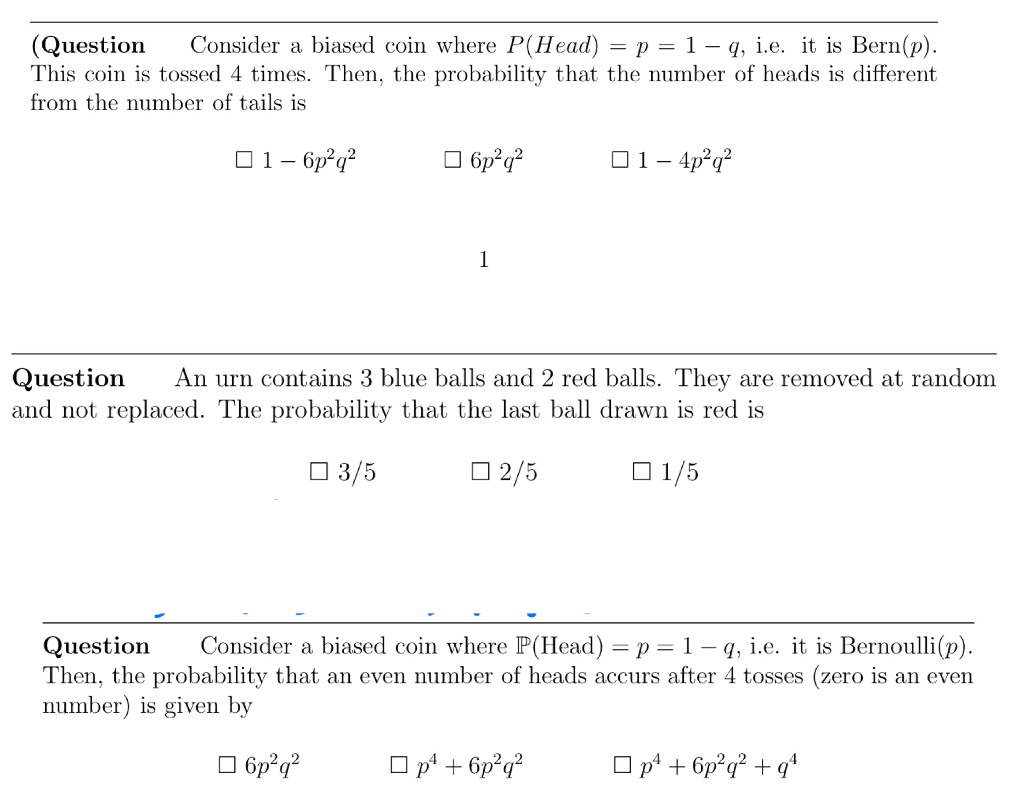(Question Consider a biased coin where P(Head) = p = 1-q, i.e. it is Bern(p) This coin is tossed 4 times. Then, the probability that the number of heads is different rom the number of tails is Question An urn contains 3 blue balls and 2 red balls. They are removed at random and not replaced. The probability that the last ball drawn is red is □ 3/5 □ 2/5 1/5 Question Consider a biased coin where P(Head)-p-1 -q, i.e. it is Bernoulli(p) Then, the probability that an even number of heads accurs after 4 tosses (zero is an even number) is given by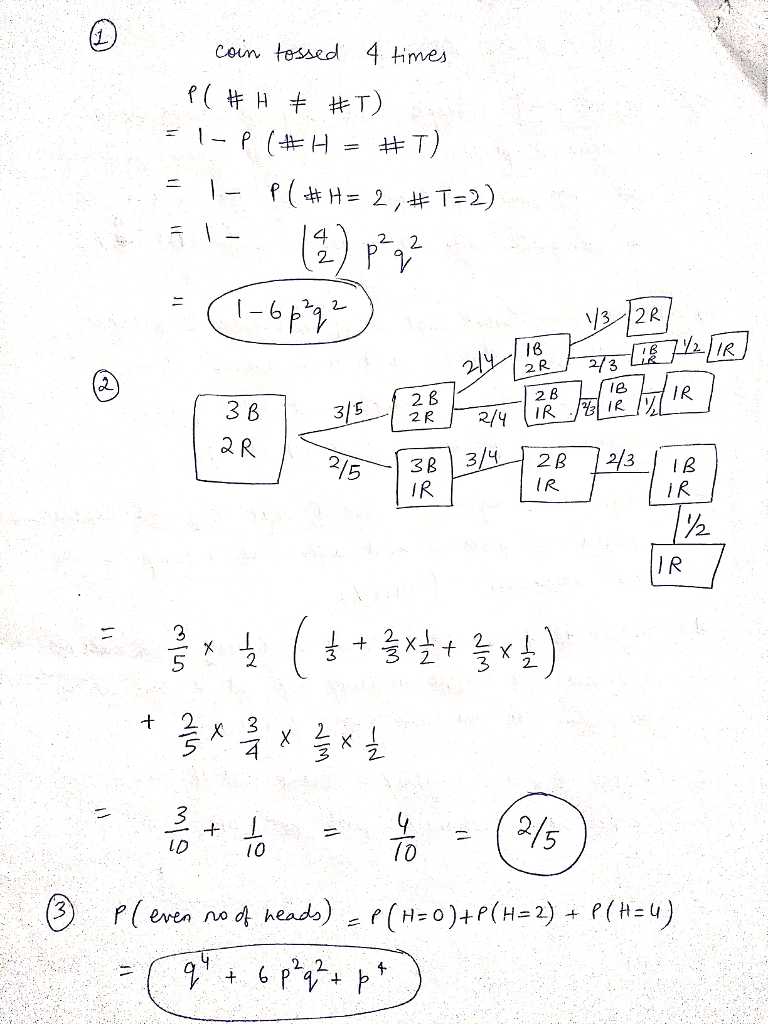#### Earn Coins

Coins can be redeemed for fabulous gifts.

Similar Homework Help Questions
• ### A biased coin is tossed n times. The probability of heads is p and the probability of tails is q and p=2q. Choose all correct statements. This is an example of a Bernoulli trial n-n-1-1-(k-1) p...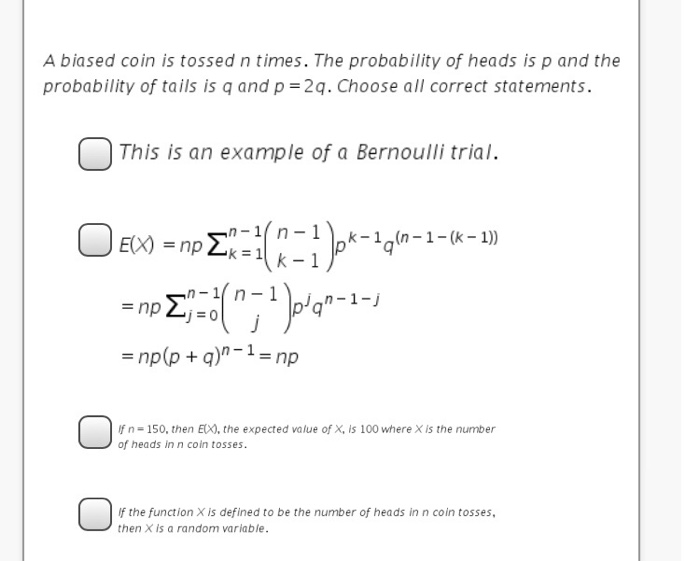A biased coin is tossed n times. The probability of heads is p and the probability of tails is q and p=2q. Choose all correct statements. This is an example of a Bernoulli trial n-n-1-1-(k-1) p'q =np(p + q)n-1 = np f n- 150, then EX), the expected value of X, is 100 where X is the number of heads in n coin tosses. f the function X is defined to be the number of heads in n coin tosses,...

• ### A biased coin is tossed until a head occurs. If the probability of heads on any...

A biased coin is tossed until a head occurs. If the probability of heads on any given toss is .4, What is the probability that it will take 7 tosses until the first head occurs? The answer i got was , (.60)^2(.40) Now for the second part it says, what is the probability that it will take 9 tosses until the second head occurs. Is the answer for this part be 9C2(.40)^2(.60)^7 or 8C1(.40)(.60)^5 I can't figure out if its...

• ### Consider a coin with probability q of landing on heads, and probability 1−q of landing on...

Consider a coin with probability q of landing on heads, and probability 1−q of landing on tails. a) The coin is tossed N times. What is the probability that the coin lands k times on heads. b) The coin is tossed 100 times, and lands on heads 70 times. What is the maximum likelihood estimate for q?

• ### A coin with probability p of heads is tossed until the first head occurs. It is...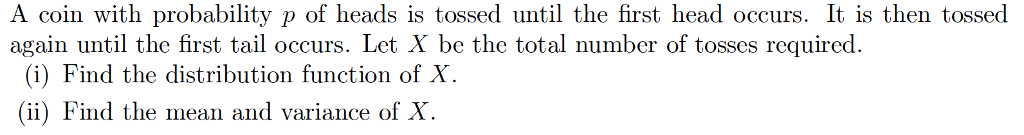A coin with probability p of heads is tossed until the first head occurs. It is then tossed again until the first tail occurs. Let X be the total number of tosses required. (i) Find the distribution function of X. (ii) Find the mean and variance of X

• ### Consider a collection of biased coins, each showing Heads with probability and Tails with probability , independently of the others

Consider a collection of biased coins, each showing Heads with probability and Tails with probability , independently of the others. The coins are tossed and all coins showing Heads are collected together and tossed again. Write down an expression for the probability mass function of the random variable representing the number of Heads that turn up in the second round of tosses. Hint: Condition on the results of the first round of tosses using the “total probability" formula.

• ### A biased coin with probability 0.6 to land on head is flipped 6 times, calculate the...

A biased coin with probability 0.6 to land on head is flipped 6 times, calculate the probability of: - exactly two heads, - at most one tail, - even number of heads.

• ### You have a biased coin, where the probability of flipping a heads is 70%. You flip...

You have a biased coin, where the probability of flipping a heads is 70%. You flip once, and the coin comes up tails. What is the expected number of flips from that point (so counting that as flip #0) until the number of heads flipped in total equals the number of tails?

• ### 1. Let p-: P(head) when a coin is tossed, and consider the hypotheses Ho : p-0.5...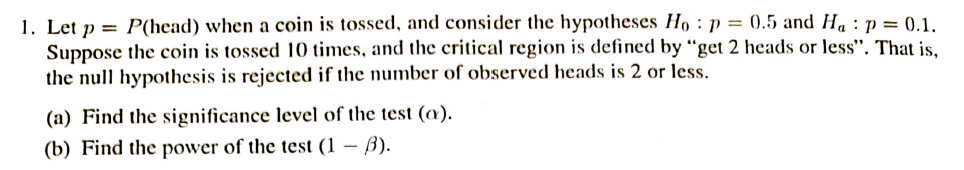1. Let p-: P(head) when a coin is tossed, and consider the hypotheses Ho : p-0.5 and H. : p-0.1. Suppose the coin is tossed 10 times, and te critcal region is defined by "get 2 heads or less". That is the null hypothesis is rejected if the number of observed heads is 2 or less. (a) Find the significance level of the test (a). (b) Find the power of the test (1 -B).

• ### Problem 4. A fair coin is tossed consecutively 3 times. Find the conditional probability P(A |...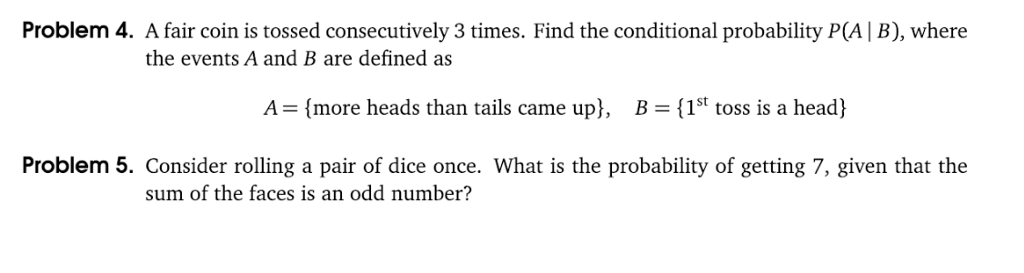Problem 4. A fair coin is tossed consecutively 3 times. Find the conditional probability P(A | B), where the events A and B are defined as A-(more heads than tails came upl, B-(1st toss is a head) 1St toss is a head Problem 5. Consider rolling a pair of dice once. What is the probability of getting 7, given that the sum of the faces is an odd number?

• ### E. A coin with probabiltiy p of heads is tossed till the first head occurs. It...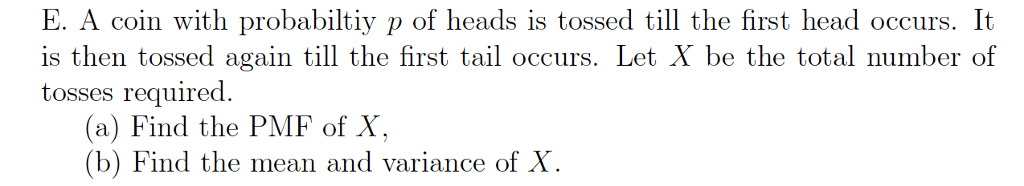E. A coin with probabiltiy p of heads is tossed till the first head occurs. It 1S is then tossed again till the first tail occurs. Let X be the total number of tosses required (a) Find the PMF of X, (b) Find the mean and variance of X